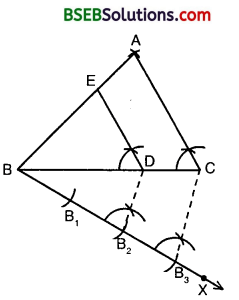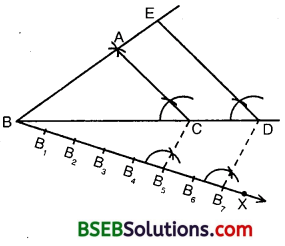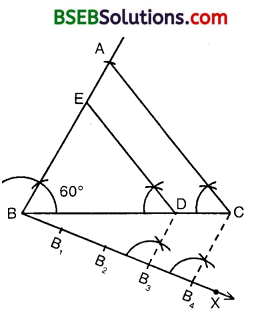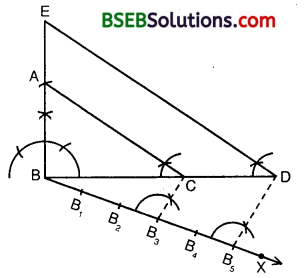# Bihar Board Class 10th Maths Solutions Chapter 11 Constructions Ex 11.1

Bihar Board Class 10th Maths Solutions Chapter 11 Constructions Ex 11.1 Textbook Questions and Answers.

## BSEB Bihar Board Class 10th Maths Solutions Chapter 11 Constructions Ex 11.1Question 1.
Draw a line segment of length 7.6 cm and divide it in the ratio 5 : 8. Measure the two parts.
Solution:
Steps of Construction :1. Draw a line segment AB = 7.6 cm.

2. Draw a ray AC making any acute angle with AB, as shown in the figure.

3. On ray AC, starting from A, mark 5 + 8 = 13 equal line segments : AA1, A1A2, A2A3, A3A4, A4A5, A5A6, A6A7, A7A8, A8A9, A9A10, A10A11, A11A12 and A12A13.

4. Join A13B.

5. From A5, draw A5P || A13B, meeting AB at P.

6. Thus, P divides AB in the ratio 5 : 8.
On measuring the two parts, we find that AP = 2.9 cm and PB = 4.7 cm (approx.).

Justification :
In ∆ ABA13, PA5 || BA13.
So, $$\frac { AP }{ PB }$$ = $$\frac{\mathrm{AA}_{5}}{\mathrm{~A}_{5} \mathrm{~A}_{13}}$$ = $$\frac { 5 }{ 8 }$$ [By BPT]
Thus, AP : PB = 5 : 8

Question 2.
Construct a triangle of sides 4 cm, 5 cm and 6 cm and then a triangle similar to it whose sides are $$\frac { 2 }{ 3 }$$ of the corresponding sides of it.
Solution:
Steps of Construction :1. Draw a line segment BC = 6 cm.

2. With B as centre and radius equal to 5 cm, draw an arc.

3. With C as centre and radius equal to 4 cm, draw an arc intersecting the previously drawn arc at A.

4. Join AB and AC. Then ∆ ABC is the required triangle.

5. Below BC, make an acute angle CBX.

6. Along BX, mark off three (bigger of the numerator and denominator) points : B1, B1 and B3 such that BB1 = B1B2 = B2B3

7. Join B3C. [Note that 3 is denominator]

8. From B2 draw B2D || B3C, meeting BC at D. [Note that 2 is numerator]

9. From D, draw DE || CA, meeting BA at E. Then, ∆ EBD is the required triangle whose sides are $$\frac { 2 }{ 3 }$$ of the corresponding sides of ∆ ABC.

Justification :
Since DE || CA, therefore
∆ ABC ~ ∆ EBD
and $$\frac { EB }{ AB }$$ = $$\frac { DE }{ CA }$$ = $$\frac { BD }{ BC }$$ = $$\frac { 2 }{ 3 }$$ [Since $$\frac { BD }{ BC }$$ = $$\frac { 2 }{ 3 }$$ ,by construction]
Hence, we get the new triangle similar to the given triangle whose sides are equal to $$\frac { 2 }{ 3 }$$ of the corresponding sides of ∆ ABC.Question 3.
Construct a triangle with sides 5 cm, 6 cm and 7 cm and then another triangle whose sides are $$\frac { 7 }{ 5}$$ of the corresponding sides of the first triangle.
Solution:
Steps of Construction :
1. With the given data, construct ∆ ABC in which BC = 7 cm, CA = 4 cm and AB = 5 cm.

2. Below BC, make an acute angle CB1.3. Along BX, mark off seven (bigger of the numerator and denominator) points : B1, B2, B3, B4, B5, B6 and B7 such that BB1 = B1B2 = B2B3 = B3B4 = B4B5 = B5B6 = B6B7

4. Join B5C. [Note that 5 is denominator]

5. From B7, draw B7D || B5C, meeting BC produced at D. [Note that 7 is numerator]

6. From D, draw DE || CA, meeting BA produced at E. Then, ∆ EBD is the required triangle whose sides are $$\frac { 7 }{ 5}$$ of the corresponding sides of ∆ ABC.

Justification :
Since DE || CA, therefore
∆ ABC ~ ∆ EBD and $$\frac { EB }{ AB }$$ = $$\frac { DE }{ CA }$$ = $$\frac { BD }{ BC }$$ = $$\frac { 7 }{ 5 }$$
Hence, we get the new triangle similar to the given triangle whose sides are equal to $$\frac { 7 }{ 5 }$$ of the corresponding sides of ∆ ABC.

Question 4.
Construct an isosceles triangle whose base is 8 cm and altitude 4 cm and then another triangle whose sides are 1$$\frac { 1 }{ 2 }$$ times the corresponding sides of the isosceles triangle.
Solution:
Steps of Construction :1. Draw BC = 8 cm.

2. Construct PQ, the perpendicular bisector of line segment BC meeting BC at M.

3. Along MP, cut off MA = 4 cm.

4. Join BA and CA. Then, ∆ ABC so obtained is the required triangle.

5. Make an acute angle CBX below BC.

6. Mark three points B1, B2 and B3 on BX such that BB1 = B1B2 = B2B3

7. Join BA2C.

8. From B3, draw B3D || B2C meeting BC produced at D.

9. From D, draw DE || CA meeting BA produced at E. Then, ∆ EBD is the required triangle.

Justification :
Since DE || CA, therefore ∆ ABC ~ ∆ EBD
and $$\frac { EB }{ AB }$$ = $$\frac { DE }{ CA }$$ = $$\frac { BD }{ BC }$$ = $$\frac { 3 }{ 2 }$$
Hence, we get the new triangle similar to the given $$\frac { 3 }{ 2 }$$ triangle whose sides are $$\frac { 3 }{ 2 }$$, i.e., 1 $$\frac { 1 }{ 2 }$$ times of the corresponding sides of the isosceles ∆ ABC.Question 5.
Draw a triangle ABC with side BC = 6 cm, AB = 5 cm and ∠ABC = 60°. Then construct a triangle whose sides are $$\frac { 3 }{ 4 }$$ of the corresponding sides of the triangle ABC.
Solution:
Steps of Construction :
1. With the given data, construct ∆ ABC in which BC 6 cm, ∠ABC = 60° and AB = 5 cm.

2. Below BC, make an acute angle CB1.

3. Along BX, mark off 4 points : B1, B2, B3 and B4 such that BB1 = B1B2 = B2B3 = B3B4.

4. Join B4C.5. From B3, draw B3D || B4C to meet BC at D.

6. From D, draw ED || AC, meeting BA at E. Then, ∆ EBD is the required triangle whose sides are $$\frac { 3 }{ 4 }$$ th of the corresponding sides of ∆ ABC.

Justification :
Since DE || CA, therefore ∆ ABC ~ ∆ EBD
and $$\frac { EB }{ AB }$$ = $$\frac { DE }{ CA }$$ = $$\frac { BD }{ BC }$$ = $$\frac { 3 }{ 4 }$$
Hence, we get the new triangle similar to the given triangle whose sides are equal to $$\frac { 3 }{ 4 }$$ of the corresponding sides of ∆ ABC.

Question 6.
Draw a triangle ABC with side BC = 7 cm, ∠B = 45% ∠A = 105°. Then construct a triangle whose sides are $$\frac { 4 }{ 3 }$$ times the corresponding sides of ∆ ABC.
Solution:
Steps of Construction :
1. With the given data, construct ∆ ABC in which BC = 7 cm, ∠B = 45°, ∠C = 180° – (∠A + ∠B).i. e., ∠C = 180° – (105° + 45°)
= 180° – 150° = 30°.

2. Below BC, make an acute ∠CBX.

3. Along BX, mark off four points : B1, B2, B3 and B4 such that BB1 = B1B2 = B2B3 = B3B4.

4. Join B3C.

5. From B4, draw B4D || B3C, meeting BC produced at D.

6. From D, draw DE || CA, meeting BA produced at E. Then, ∆ EBD is the required triangle whose sides are $$\frac { 4 }{ 3 }$$ times of the corresponding sides of ∆ ABC.

Justification :
Since DE || CA, therefore ∆ ABC ~ ∆ EBD
and $$\frac { EB }{ AB }$$ = $$\frac { DE }{ CA }$$ = $$\frac { BD }{ BC }$$ = $$\frac { 4 }{ 3 }$$
Hence, we get the new triangle similar to the given triangle whose sides are equal to $$\frac { 4 }{ 3 }$$ times of the corresponding I sides of ∆ ABC.Question 7.
Draw a right triangle in which the sides (other than hypotenuse) are of lengths 4 cm and 3 cm. Then construct another triangle whose sides are $$\frac { 5 }{ 3 }$$ times the corresponding sides of the given triangle.
Solution:
Steps of Construction :
1. With given data, construct ∆ ABC in which BC = 4 cm, ∠B = 90° and BA = 3 cm.

2. Below BC, make an acute angle CBX.

3. Along BX, mark off four points : B1, B2, B3 and B4 such that BB1 = B1B2 = B2B3 = B3B4.

4. Join B3C.5. From B5, draw B5D || B3C, meeting BC produced at D.

6. From D, draw DE || CA, meeting BA produced at E.
Then, EBD is the required triangle whose sides are $$\frac { 5 }{ 3 }$$ times of the corresponding sides of ∆ ABC.

Justification :
Since DE || CA, therefore ∆ ABC ~ ∆ EBD
and $$\frac { EB }{ AB }$$ = $$\frac { DE }{ CA }$$ = $$\frac { BD }{ BC }$$ = $$\frac { 5 }{ 3 }$$
Hence, we get the new triangle similar to the given triangle whose sides are equal to $$\frac { 5 }{ 3 }$$ times of the corresponding sides of ∆ ABC.